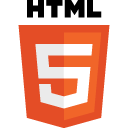## Learning HTML5 Canvas with JavaScript – step by stepWe’ve already published a basic course of HTML5, with a solid foundation for learning (or reminders). With such basics we can dive into more advanced (and interesting) topics. Learning HTML5 Canvas can be disassembled into smaller parts, and to handle them step by step.

#### Learning HTML5 Canvas

Learning goes great through the implementation of specific examples, so… Here we go, with a simple way to start doing cool things in HTML5.

Let’s create a template, where we will run the test code:

```<!DOCTYPE html>
<html lang="en">
<meta charset="utf-8">

<script type="application/javascript">
function draw() {
var canvas = document.getElementById("canvas");
var ctx = canvas.getContext("2d");

// the code goes HERE ...

}

</script>

<canvas id="canvas" width="500" height="300"
style="border: solid 1px;"></canvas>
</body>

</html>
```

At the very beginning: path and drawing straight lines. We use two functions:

moveTo(x, y) – defines the starting point of the line,

lineTo(x, y) – the ending point.

The line will be created between these two points.

Example #1 – drawing a line:

```// ...
ctx.moveTo(0, 0);
ctx.lineTo(300, 150);

// set line color
ctx.strokeStyle = '#0000ff';
ctx.stroke();
```

We define parameters of the line (e.g. color). After executing this code, we should get following result:

Simple. So now let’s try to draw a rectangle and a circle.

To draw a rectangle we need the rect(x, y, w, h) method.

Example #2 – drawing a rectangle on HTML5 Canvas:

```// ...
ctx.beginPath();
ctx.rect(20, 20, 250, 150);
ctx.fillStyle = '#000000';
ctx.fill();
ctx.lineWidth = 3;
ctx.strokeStyle = '#0000ff';
ctx.stroke();
```

We defined basic parameters (color, border) and the effect is:

To draw a circle, the arc(x, y, r, start, stop) method is used.

Example #3 – drawing a circle:

```// #1
ctx.beginPath();
ctx.arc(100, 100, 50, 0, 2 * Math.PI);
ctx.stroke();

// #2
ctx.beginPath();
ctx.arc(380, 100, 50, 0, 2 * Math.PI);
ctx.stroke();
```

In this example, we draw two circles that look like this:

HTML5 Canvas and text

The Canvas wouldn’t be so cool without the possibility of text writing. Fortunately, it’s quite simple.

The most important methods and parameters adjustable for the context, in this case are:

font – here we define font settings for our text,

fillText(text, x, y) – this method draws a filled text at a given position,

strokeText(text, x, y) – draws text without filling.

Example #4 – our text on HTML5 Canvas:

```// ...
ctx.font = "32px Verdana";
ctx.fillText("Learning HTML5 Canvas", 5, 40);

```

An example above displays the filled text, while the following code:

```// ...
ctx.font = "32px Verdana";
ctx.strokeText("Learning HTML5 Canvas", 5, 40);
```

displays text without filling:

HTML5 Canvas and creating a gradient

Gradients are used to fill other graphic objects (shapes), usually where we don’t want solid colors.

We can create a linear gradient:

or “circular”:

Then specify the color transitions (color stops). For this we use the addColorStop() method.

```// ...
var g = ctx.createLinearGradient(0, 0, 200, 0);

ctx.fillStyle = g;
ctx.fillRect(10, 10, 200, 100);

```

And here is the result:

A simple chart in HTML5

Simply define the input and loop that passes over them, and draws a sufficiently high stakes.

Example #6 – bar graph:

```// ...

// data
var inputData = [99, 60, 20, 10, 10, 70, 125, 100];

var canvasWidth = 500;

// the place from where we start drawing
var x_from = 40;

// the distance between the posts
var step = Math.round(canvasWidth / inputData.length) - 5;

for (var i = 0; i < inputData.length; i++) {
// the x position of the next post
var x_next = x_from + (i * step) - 20;

// drawing a post...
ctx.fillStyle = "rgba(50, 150, 250, 1)";
ctx.fillRect(x_next, 150, 40, -inputData[i]);

ctx.fillStyle = "rgba(10, 10, 50, 0.4)";
ctx.fillRect(x_next, 150, 45, -inputData[i]);
}

```

And here is the result:

And for the curious – recommended resources:

– Canvas reference on W3Schools:

http://www.w3schools.com/tags/ref_canvas.asp

– Practical examples with a brief commentary:

http://www.tutorialspoint.com/html5/html5_canvas.htm

#### Learning HTML5 Canvas – summary

This concludes today’s article. More advanced examples coming soon. In the end, learning is also a constant practice and experimentation.

Thank you and have fun with HTML5 Canvas!

Both comments and pings are currently closed.

### One Response to “Learning HTML5 Canvas with JavaScript – step by step”

1.DirectCode says: